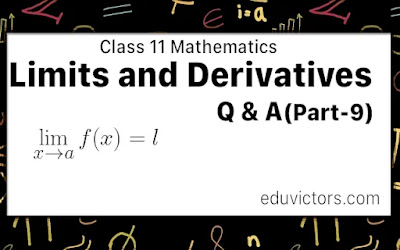# THEOREMS ON DIFFERENTIATION

CBSE Class 11 - Mathematics - Limits and Derivatives Part-9

You have learnt about finding derivatives using the first principle in part-7 and part-8. Let us proceed further. Here is a summary of theorems on differentiation.

Theorem I :

$\frac{\mathrm{d} }{\mathrm{d} x}[kf (x)] = k d/dx [f (x)]$, where k is a constant

Theorem II :

$\frac{\mathrm{d} }{\mathrm{d} x}\left [ f_{1}(x)\pm f_{2}(x)\pm f_{3}(x)\pm ....... \right ] = \frac{\mathrm{d} }{\mathrm{d} x}[f_{1}(x)]\pm \frac{\mathrm{d} }{\mathrm{d} x}[f_{2}(x)]\pm \frac{\mathrm{d} }{\mathrm{d} x}[f_{3}(x)]\pm .......$

Theorem III :

$\frac{\mathrm{d} }{\mathrm{d} x}[f(x)\cdot g(x)] = f(x)\frac{\mathrm{d} }{\mathrm{d} x}[g(x)] + g(x)\frac{\mathrm{d} }{\mathrm{d} x}[f(x)]$

Theorem IV :

$\frac{\mathrm{d} }{\mathrm{d} x}\left [ \frac{f(x)}{g(x)} \right ]=\frac{g(x)d/dx[f(x)]-f(x)d/dx[g(x)]}{[g(x)]^{2}}$

Theorem V :

Derivative of the function of the function: If 'y' is a function of 't' and 't' is a function of 'x' then,

$\frac{\mathrm{d} y}{\mathrm{d} x}=\frac{\mathrm{d} y}{\mathrm{d} t}\cdot \frac{\mathrm{d} t}{\mathrm{d} x}$

Theorem VI :

Derivative of parametric equations: if x = f (t), y = ψ (t) then $\frac{\mathrm{d} y}{\mathrm{d} x}=\frac{dy/dt}{dx/dt}$

Theorem VII :

Derivative of a function with respect to another function: If f (x) and g (x) are two functions of a variable x, then

$\frac{\mathrm{d} [f(x)]}{\mathrm{d} [g(x)]}=\frac{\mathrm{d} }{\mathrm{d} x}f(x)/\frac{\mathrm{d} }{\mathrm{d} x}g(x)$

Theorem VIII :

$\frac{\mathrm{d} y}{\mathrm{d} x}\cdot \frac{\mathrm{d} x}{\mathrm{d} y}=1$

Theorem IX :

The general power rule: If y = f [g(x)] = [g (x)]n then the chain rule yields

$\frac{\mathrm{d} y}{\mathrm{d} x}=\frac{\mathrm{d} y}{\mathrm{d} u}\cdot \frac{\mathrm{d} u}{\mathrm{d} x}=nu^{n-1}\cdot \frac{\mathrm{d} }{\mathrm{d} x}g(x)=n[g(x)]^{n-1}\cdot \frac{\mathrm{d} }{\mathrm{d} x}g(x)$

If n is any real number and g' (x) exists, then $\frac{\mathrm{d} }{\mathrm{d} x}[g(x)]^{n}=n[g(x)]^{n-1}\cdot \frac{\mathrm{d} }{\mathrm{d} x}g(x)$

You might think of the general power rule as power in front of the function, reduce the power of function by one, times the derivative of a function. A common error is to ignore the $\frac{\mathrm{d} }{\mathrm{d} x}g(x)$ term in the general power formula.

Ch 13 Limits and Derivatives (Q & A) Part-5

Ch 13 Limits and Derivatives (Q & A) Part-6

Ch 13 Limits and Derivatives (Q & A) Part-7